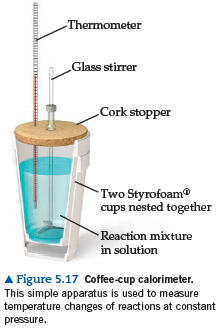# Problem: A coffee-cup calorimeter of the type shown in Figure 5.17 contains 150.0 g of water at 25.1°C. A 121.0-g block of copper metal is heated to 100.4°C by putting it in a beaker of boiling water. The specific heat of Cu(s) is 0.385 J/g•K. The Cu is added to the calorimeter, and after a time the contents of the cup reach a constant temperature of 30.1°C. (c) The difference between your answers for (a) and (b) is due to heat loss through the Styrofoam® cups and the heat necessary to raise the temperature of the inner wall of the apparatus. The heat capacity of the calorimeter is the amount of heat necessary to raise the temperature of the apparatus (the cups and the stopper) by 1 K. Calculate the heat capacity of the calorimeter in J/K.

###### FREE Expert Solution
90% (275 ratings)
###### Problem Details

A coffee-cup calorimeter of the type shown in Figure 5.17 contains 150.0 g of water at 25.1°C. A 121.0-g block of copper metal is heated to 100.4°C by putting it in a beaker of boiling water. The specific heat of Cu(s) is 0.385 J/g•K. The Cu is added to the calorimeter, and after a time the contents of the cup reach a constant temperature of 30.1°C. (c) The difference between your answers for (a) and (b) is due to heat loss through the Styrofoam® cups and the heat necessary to raise the temperature of the inner wall of the apparatus. The heat capacity of the calorimeter is the amount of heat necessary to raise the temperature of the apparatus (the cups and the stopper) by 1 K. Calculate the heat capacity of the calorimeter in J/K.